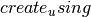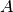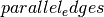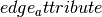Warning

This documents an unmaintained version of NetworkX. Please upgrade to a maintained version and see the current NetworkX documentation.

# from_scipy_sparse_matrix¶

from_scipy_sparse_matrix(A, parallel_edges=False, create_using=None, edge_attribute='weight')[source]

Creates a new graph from an adjacency matrix given as a SciPy sparse matrix.

Parameters: A (scipy sparse matrix) – An adjacency matrix representation of a graph parallel_edges (Boolean) – If this is True,is a multigraph, andis an integer matrix, then entry (i, j) in the matrix is interpreted as the number of parallel edges joining vertices i and j in the graph. If it is False, then the entries in the adjacency matrix are interpreted as the weight of a single edge joining the vertices. create_using (NetworkX graph) – Use specified graph for result. The default is Graph() edge_attribute (string) – Name of edge attribute to store matrix numeric value. The data will have the same type as the matrix entry (int, float, (real,imag)).

Notes

Ifis an instance of networkx.MultiGraph or networkx.MultiDiGraph,is True, and the entries ofare of type int, then this function returns a multigraph (of the same type as) with parallel edges. In this case,will be ignored.

Ifis an undirected multigraph, then only the edges indicated by the upper triangle of the matrixwill be added to the graph.

Examples

>>> import scipy.sparse
>>> A = scipy.sparse.eye(2,2,1)
>>> G = nx.from_scipy_sparse_matrix(A)


Ifis a multigraph and the matrix has only integer entries, the entries will be interpreted as weighted edges joining the vertices (without creating parallel edges):

>>> import scipy
>>> A = scipy.sparse.csr_matrix([[1, 1], [1, 2]])
>>> G = nx.from_scipy_sparse_matrix(A, create_using=nx.MultiGraph())
>>> G
{0: {'weight': 2}}


Ifis a multigraph and the matrix has only integer entries butis True, then the entries will be interpreted as the number of parallel edges joining those two vertices:

>>> import scipy
>>> A = scipy.sparse.csr_matrix([[1, 1], [1, 2]])
>>> G = nx.from_scipy_sparse_matrix(A, parallel_edges=True,
...                                 create_using=nx.MultiGraph())
>>> G
{0: {'weight': 1}, 1: {'weight': 1}}